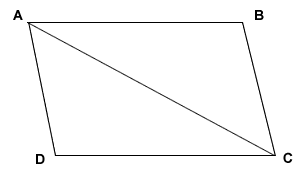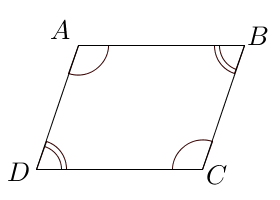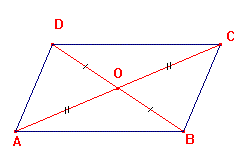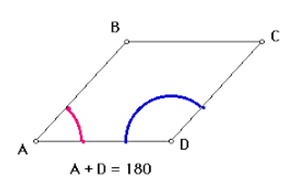# What are the properties of a parallelogram?

Property 1:

Opposite sides of a parallelogram are congruent.In the above parallelogram, AB = CD and AD = BC

Property 2:

Opposite angles of a parallelogram are congruent.In the above parallelogram,  ∠A = ∠C   and  ∠B = ∠D

Property 3:

The diagonals of a parallelogram bisect each other.Here, AC bisects BD at the intersection point, O.

Property 4:

Consecutive angles are supplementary or add up to 180 degrees.In the above parallelogram,

∠A + ∠B = 180°

∠B + ∠C = 180°

∠C + ∠D = 180°

∠D + ∠A = 180°

Updated on: 10-Oct-2022

39 Views